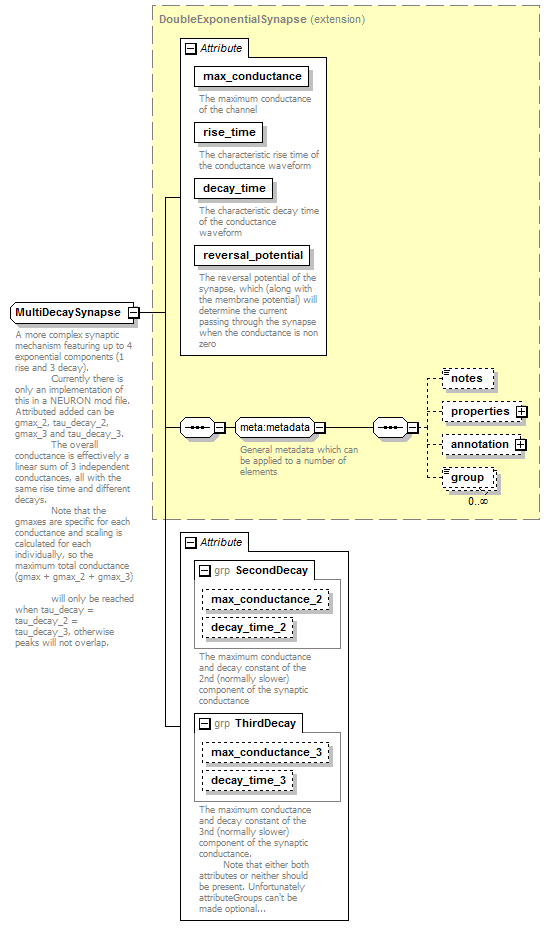# MultiDecaySynapse

 ```A more complex synaptic mechanism featuring up to 4 exponential components (1 rise and 3 decay). Currently there is only an implementation of this in a NEURON mod file. Attributed added can be gmax_2, tau_decay_2, gmax_3 and tau_decay_3. The overall conductance is effectively a linear sum of 3 independent conductances, all with the same rise time and different decays. Note that the gmaxes are specific for each conductance and scaling is calculated for each individually, so the maximum total conductance (gmax + gmax_2 + gmax_3) will only be reached when tau_decay = tau_decay_2 = tau_decay_3, otherwise peaks will not overlap.```

## Model## Attributes

QName Type Fixed Default Use Inheritable Annotation
decay_time TimeConstantValue required
 `The characteristic decay time of the conductance waveform`
decay_time_2 TimeConstantValue optional
decay_time_3 TimeConstantValue optional
max_conductance ConductanceValue required
 `The maximum conductance of the channel`
max_conductance_2 ConductanceValue optional
max_conductance_3 ConductanceValue optional
reversal_potential VoltageValue required
 `The reversal potential of the synapse, which (along with the membrane potential) will determine the current passing through the synapse when the conductance is non zero`
rise_time TimeConstantValueIncZero required
 `The characteristic rise time of the conductance waveform`

## Used By

 Element SynapseType/multi_decay_syn Complex Types FacDepSynapse, StdpSynapse

## Source

 ``` A more complex synaptic mechanism featuring up to 4 exponential components (1 rise and 3 decay). Currently there is only an implementation of this in a NEURON mod file. Attributed added can be gmax_2, tau_decay_2, gmax_3 and tau_decay_3. The overall conductance is effectively a linear sum of 3 independent conductances, all with the same rise time and different decays. Note that the gmaxes are specific for each conductance and scaling is calculated for each individually, so the maximum total conductance (gmax + gmax_2 + gmax_3) will only be reached when tau_decay = tau_decay_2 = tau_decay_3, otherwise peaks will not overlap. Extends DoubleExponentialSynapse The maximum conductance and decay constant of the 2nd (normally slower) component of the synaptic conductance The maximum conductance and decay constant of the 3nd (normally slower) component of the synaptic conductance. Note that either both attributes or neither should be present. Unfortunately attributeGroups can't be made optional... ```